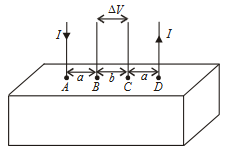#### For current entering at A, the electric field at a distance r  from A  is Directions : Consider a block of conducting material of resistivityshown in the figure. Current I enters at A    and leaves from  D  . We apply superposition principle to find voltagedeveloped between  B and C The calculation is done in the following steps:(i)    Take current  I   entering from  A  and assume it to spread over a hemispherical surface in the block.(ii)     Calculate field E(r)  at distance r   from  A  by using Ohm’s lawWhere J is the current per unit area at r(iii)     From the r  dependence of E (r) obtain the potential  V (r) at r (iv)     Repeat (i),(ii) and (iii) for current  I leaving D and superpose results for A and B Option 1)Option 2)Option 3)Option 4)As we learnt in

If current density is uniform -- whereinCurrent densityNormal cross-section

Current density,'Option 1)This option is incorrect.

Option 2)This option is incorrect.

Option 3)This option is incorrect.

Option 4)This option is correct.

#### Plabita